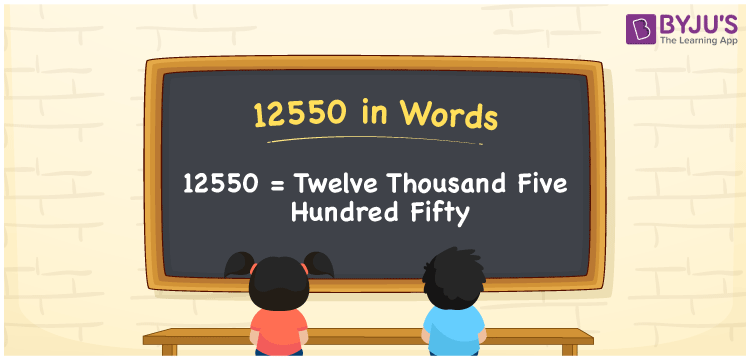# 12550 in Words

The word form of the number 12550 is expressed as Twelve Thousand Five Hundred Fifty. For instance, the cost of a dining table is Rs. 12550, then you can write, “The cost of a dining table is Rupees Twelve Thousand Five Hundred Fifty”. We know 12550is a cardinal number since it shows an exact value. The place value table assists students to write the numbers to word form easily. In this article, let us learn how to write the number 12550 in words and its interesting facts.

 12550 in Words Twelve Thousand Five Hundred Fifty Twelve Thousand Five Hundred Fifty in numerical form 12550

## 12550 in English Words

It is essential to learn the English language as it is widely used in education. Hence, numbers in words are formed using the letters of the English alphabet. Thus, we can spell and write the number 12550 in words as Twelve Thousand Five Hundred Fifty.## How to Write 12550 in Words?

With the help of a place value system, we can convert the number 12550 in words with ease. The place value chart for the number 12550 is as follows.

 Ten-Thousands Thousands Hundreds Tens Ones 1 2 5 5 0

Hence, we can write the expanded form as:

1 x Ten Thousand + 2 x Thousand + 5 x Hundred + 5 x Ten + 0 x One

= 1 x 10000 + 2 x 1000 + 5 x 100 + 5 x 10 + 0 x 1

= 10000 + 2000 + 500 + 50 + 0

= 10000 + 2000 + 500 + 50

= 12550

= Twelve Thousand Five Hundred Fifty

Hence, 12550 in words is written as Twelve Thousand Five Hundred Fifty

Interesting way of writing 12550 in words

1 = One

12 = Twelve

125 = One Hundred and Twenty-Five

1255 = One Thousand Two Hundred Fifty-Five

12550 = Twelve Thousand Five Hundred Fifty

Thus, the word form of the number 12550 is Twelve Thousand Five Hundred Fifty

12550 is a natural number that precedes 12551 and succeeds 12549

• 12550 in words – Twelve Thousand Five Hundred Fifty
• Is 12550 an odd number? – No
• Is 12550 an even number? – Yes
• Is 12550 a perfect square number? – No
• Is 12550 a perfect cube number? – No
• Is 12550 a prime number? – No
• Is 12550 a composite number? – Yes

## Frequently Asked Questions on 12550 in Words

Q1

### How to write 12550 in words?

12550 in words is Twelve Thousand Five Hundred Fifty.
Q2

### Simplify 11000 + 1550, and express in words.

Simplifying 11000 + 1550, we get 12550. Hence, the number 12550 in words is Twelve Thousand Five Hundred Fifty.
Q3

### Is 12550 a prime number?

No, the number 12550 is not a prime number.